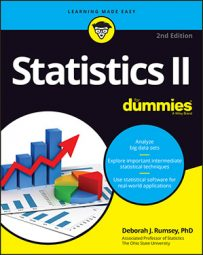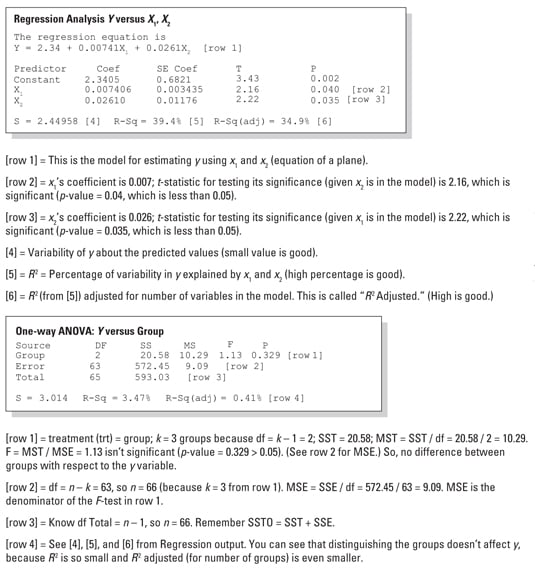##### Statistics II For DummiesStatistics II elaborates on Statistics I and moves into new territories, including multiple regression, analysis of variance (ANOVA), Chi-square tests, nonparametric procedures, and other key topics. Knowing which data analysis to use and why is important, as is familiarity with computer output if you want your numbers to give you dependable results.

## How to determine which data analysis to use in Statistics II

Statistics II is often about data analysis, and the trick is to know when to use which analysis method. The following table helps you compare, contrast, and decide what data analysis to use and when. Use it for an easy reference and to review for exams.

Analysis Purpose When It’s Used
Simple linear regression Use x to estimate y, using a line Response variable y quantitative; constant
variance across x, which is quantitative
Multiple regression Use multiple x variables (x, i = 1 . . . ,
k) to estimate y using a plane
y is quantitative; normal distribution for each
xi combination with constant variance
Nonlinear regression Use x to estimate y using a curve y is quantitative; normal distribution; constant
variance across x
Logistic regression Use x to estimate p = probability of success of
y
y is a yes/no variable with success p
One-way ANOVA Compare two population means using one factor y is quantitative; factor is x
Tukey’s test Multiple comparisons Confidence intervals for all pairs of means; keeps error rates
low
Fisher’s LSD test Multiple comparisons Confidence intervals for all pairs of means; overall error rate
higher than Tukey’s
Scheffe’s method Multiple comparisons Looks at linear combinations of means, not just pairs
Bonferroni adjustment Multiple comparisons All pairs of t-tests adjusted for number of tests
Dunnetts’s test Multiple comparisons Experiments; compares treatment versus control only
Student Newman-Keuls test (SNK) Multiple comparisons Stepwise approach, comparing pairs ordered from smallest to
largest
Duncan’s multiple range test (MRT) Multiple comparisons Adjusts SNK test for more power
Two-way ANOVA Compare more than two population means, using two factors plus
interaction
y is quantitative; factors are (x1,
x
2)
Chi-square tests Test independence of two variables or goodness-of-fit for one
qualitative variable
All variables qualitative
Sign/Signed rank tests Test one population median y is quantitative or ordinal (based on ranks)

## Computer output for Statistics II

If you’re taking Statistics II, you’re likely to face questions on computer output for multiple regression and ANOVA. Professors like to give output on exams and ask you to interpret it. Sometimes they leave empty spaces and ask you to fill them in using the info given. Reviewing the following output analyses can help.